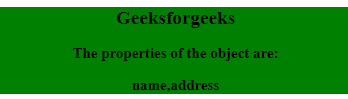# JavaScript ES5 Object Methods

The ES5 Object method in javascript is used to find more methods to interact with the objects.
The ES5 Object method can do:

• prevents enumeration
• manipulation
• deletion
• prevent addition of new features
• getters and setters

Syntax:

`Object.defineProperty(object, property, {value : value})`

The following Meta Data value can be true or false:

• writable
• enumerable
• configurable

Example to get all properties:

 ` ` `<``html``> ` ` `  `<``body``> ` `    ``<``div` `style``=``"background-color: green;"``> ` `        ``<``center``> ` `            ``<``h2``>Geeksforgeeks ` `            ``<``h3``>The properties of the object are: ` ` `  `            ``<``h3` `id``=``"demo"``> ` `        `` ` `    `` ` ` `  `    ``<``script``> ` `        ``var person = { ` `            ``name: "xyz", ` `            ``address: "noida", ` `            ``language: "hindi" ` `        ``} ` `        ``// Change Property ` `        ``Object.defineProperty(person,  ` `            ``"language", { enumerable: false }); ` ` `  `        ``document.getElementById("demo").innerHTML ` `            ``= Object.getOwnPropertyNames(person); ` `    `` ` ` ` ` `  ` `Example to get enumerable properties:

 ` ` `<``html``> ` ` `  `<``body``> ` `    ``<``div` `style``=``"background-color: green;"``> ` `        ``<``center``> ` `            ``<``h2``>Geeksforgeeks ` `            ``<``h3``>The properties of the object are: ` ` `  `            ``<``h3` `id``=``"demo"``> ` `        `` ` `    `` ` ` `  `    ``<``script` `type``=``"text/javascript"``> ` `        ``var person = { ` `            ``name: "xyz", ` `            ``address: "noida", ` `            ``language: "hindi" ` `        ``} ` ` `  `        ``// Change Property ` `        ``Object.defineProperty(person,  ` `            ``"language", { enumerable: false }); ` ` `  `        ``document.getElementById("demo") ` `            ``.innerHTML = Object.keys(person); ` `    `` ` ` ` ` `  ` `` ` `<``html``> ` ` `  `<``body``> ` `    ``<``div` `style``=``"background-color: green;"``> ` `        ``<``center``> ` `            ``<``h2``>Geeksforgeeks ` `            ``<``h3``> ` `                ``The mobno property is added and  ` `                ``the value of that property is: ` `            `` ` ` `  `            ``<``h3` `id``=``"demo"``> ` `        `` ` `    `` ` ` `  `    ``<``script``> ` `        ``var person = { ` `            ``name: "xyz", ` `            ``address: "noida", ` `            ``language: "hindi" ` `        ``} ` ` `  `        ``Object.defineProperty(person,  ` `            ``"mobno", { value: "979889xxxx" }); ` ` `  `        ``// Display Properties ` `        ``document.getElementById("demo") ` `            ``.innerHTML = person.mobno; ` `    `` ` ` ` ` `  ` `